# 第04次作业-树

## 1.学习总结

### 1.1树结构思维导图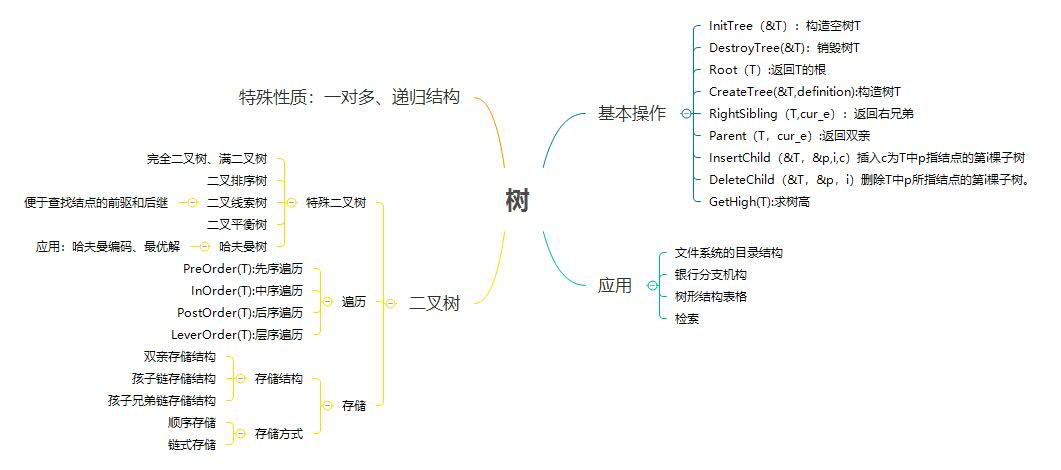### 1.2 树结构学习体会

• 对树的认识
树是一个一对多的递归结构，包含有序树和无序树。无序树中的二叉树为重要学习部分。
二叉树的度最大为2，可用链式和顺序存储方式存储。包含二叉排序树、线索二叉树、哈夫曼树、平衡二叉树等特殊二叉树。
二叉树的遍历包含先序遍历、中序遍历、后序遍历和层次遍历。
• 学习过程中遇到的困难
对树的性质和术语理解不清
对平衡二叉树的LR LL RL RR 等具体操作不理解
在哈夫曼树计算 WPL 时曾忘记他是二叉树
不能很好的利用树的递归思想
代码不会写是一个很大的困难了
• 树结构可以解决的问题
文件系统的目录结构
相比数组和链表查找速度更快，为O(logn)

## 2.PTA实验作业

### 2.1 题目1：7-3 jmu-ds-二叉树层次遍历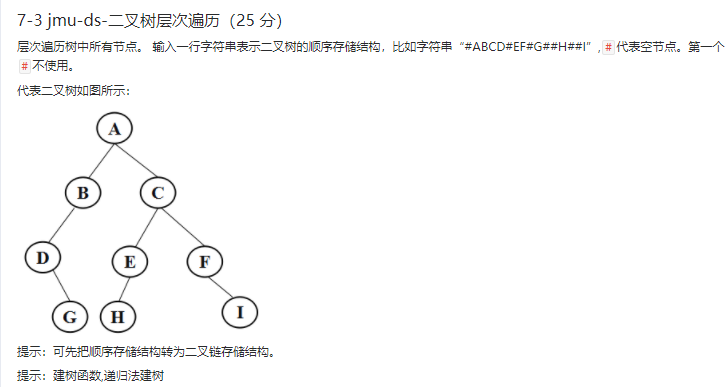### 2.2 设计思路

void LeverOrder( BTNode *b)
/* 层序遍历 */

if b 不为空
then while 队不为空
队首元素 ← p
DeQueue ( qu )
输出 p->data
if p->lchild 不为空
then Enqueue( p->lchild )
if p->lchild 不为空
then Enqueue( p->lchild )
end

BTree trans(string str,int i)
/*递归建立二叉树 */

if i > str.size()
then 返回空
if str[i] = '#'
then 返回空
b->data ← str[i]



### 2.3 代码截图

• 结构体定义及函数声明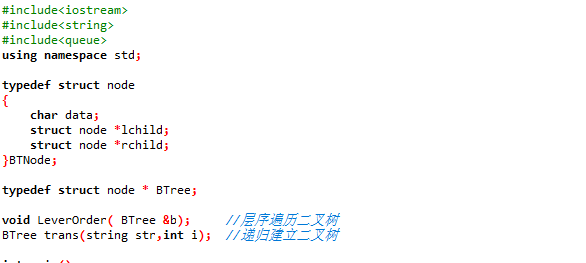• 主函数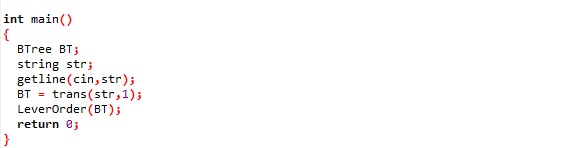• 二叉树层序遍历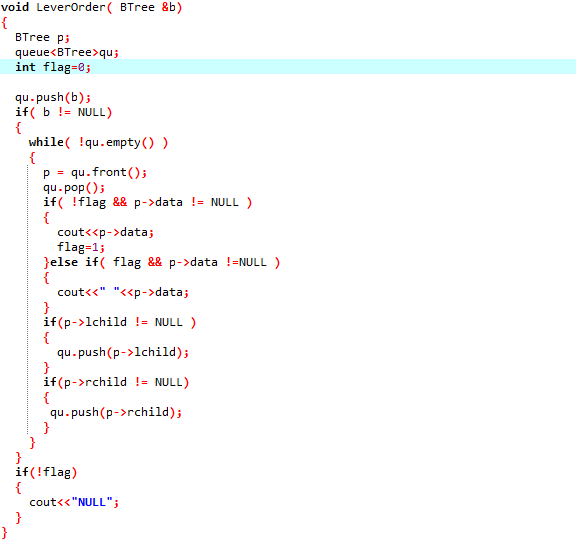• **递归建立二叉树 **### 2.4 PTA提交列表说明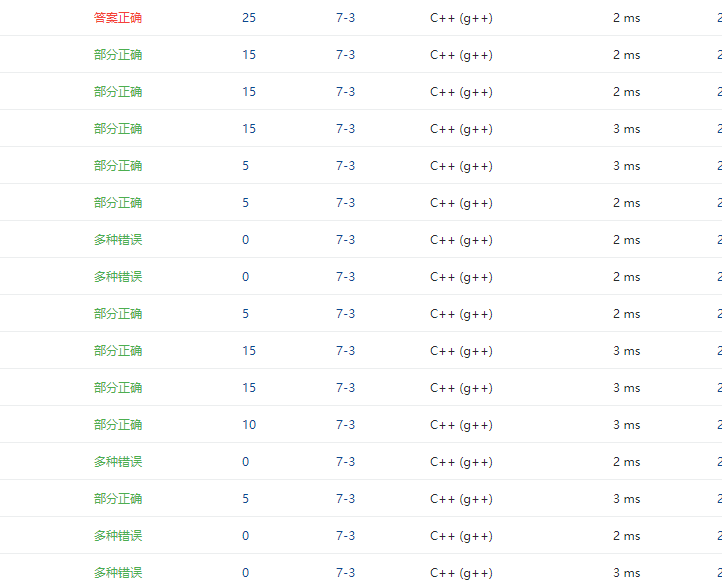• 段错误：没有考虑到 str[i] 为空的情况
解决方法：添加代码if(i>str.size()) { return NULL; }
• 答案错误：队列不为空没有包含在 b 不为空的条件下
解决方法：询问同学后解决

### 2.1 题目2：7-2 根据后序和中序遍历输出先序遍历### 2.2 设计思路

BTNode *CreateBTree( int n,int *in,int *post)
/*利用后序和中序递归建树 */

if n < 0
then 返回 空
BT->data ← post[n-1]

for i=0 to n
if in[i] = BT->data
then break
end



### 2.3 代码截图

• 结构体定义及函数声明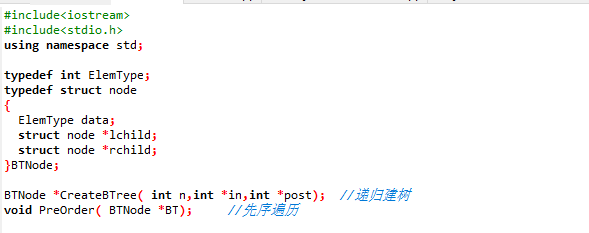• 主函数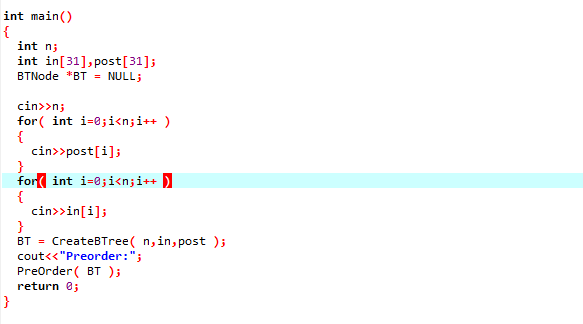• 利用后序和中序递归建树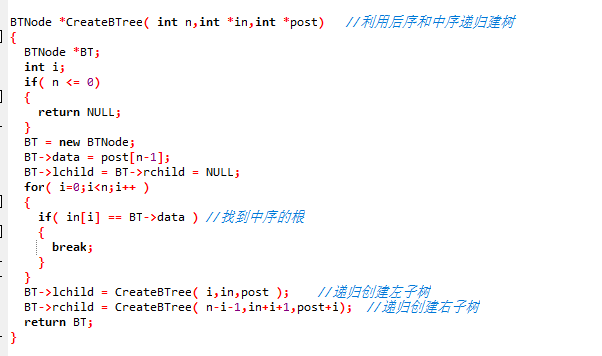### 2.4 PTA提交列表说明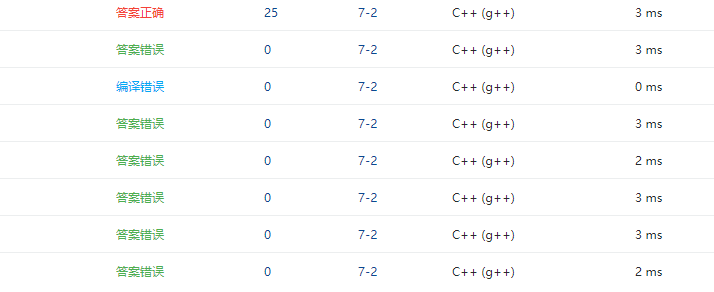• 答案错误：忘记了数组只存储到 n-1 个位置的性质，在调用函数创建右子树时传入的 n 写成了 n-i
解决方法：修改代码 CreateBTree( n-i-1,in+i+1,post+i)
• 段错误：没有将 BT 的左右子树置为空
解决方法：添加代码BT->lchild = BT->rchild = NULL;

### 2.1 题目3：6-4 jmu-ds-表达式树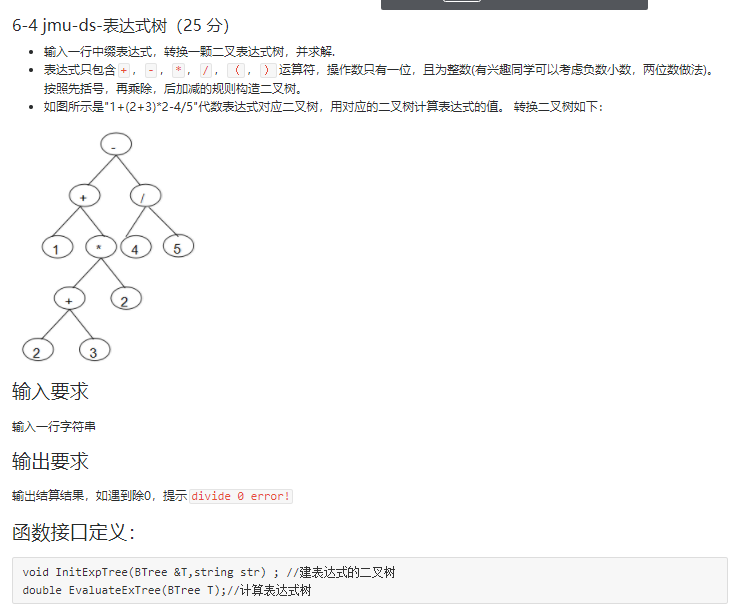### 2.2 设计思路

/*建表达式的二叉树*/

while str[i] 不为空
if str[i] 为操作符
then 创建结点并入栈
else        /*判断当前运算符和栈顶运算符的优先级*/
then if optr栈 栈顶运算符优先级高
then 出 optr栈 元素并创建结点 T
T->rchild ←出 digit栈 元素,  T->lchild ←出 digit栈 元素
elseif optr栈 栈顶元素优先级低
then 入栈当前运算符
else
出 optr 栈运算符
end

while optr栈顶元素 ≠ ‘#’
出 optr栈 元素并创建结点 T
T->rchild ←出 digit栈 元素,  T->lchild ←出 digit栈 元素
end
T ← digit栈 栈顶元素

double EvaluateExTree(BTree T)
/* 计算表达式树 */

if T 为叶子结点
then 返回 T 所指的数值
else
a ← EvaluateExTree( T->lchild )
b ← EvaluateExTree( T->rchild )
用 T 的运算符来处理 a b


### 2.3 代码截图

• 建表达式的二叉树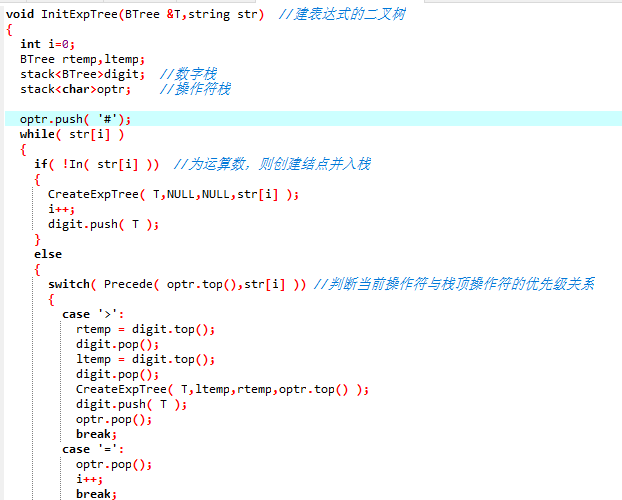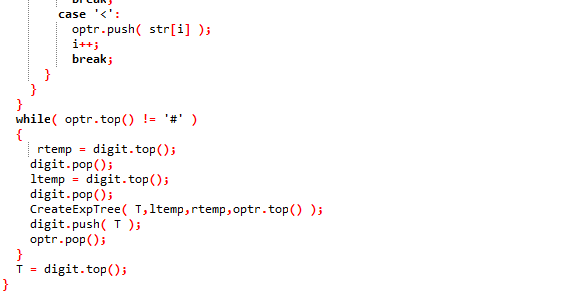• 计算表达式树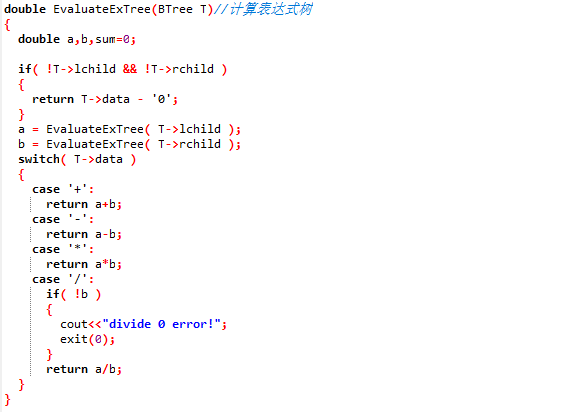### 2.4 PTA提交列表说明• 段错误：在调用优先级判断函数时写成Precede( str[i],optr.top() )
解决方法：注意到题目中的判断优先级函数中没有 t1 为 ‘）’ 的情况，修改代码为Precede( optr.top(),str[i] )
• 答案错误：没有在一开始让 ‘#’ 入栈
解决方法：后面询问同学知道，需要做这样一个标记

### 3.1 PTA排名截图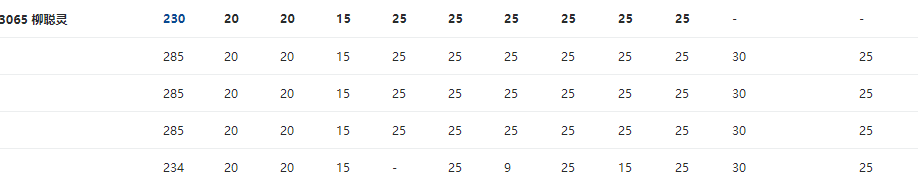### 4.阅读代码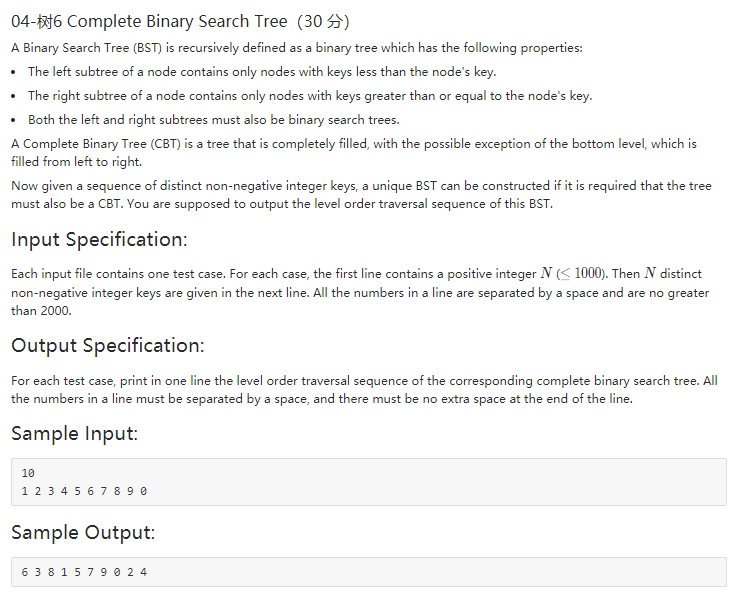#include<iostream>
#include<vector>
#include<algorithm>
using namespace std;

int N;
int pos=0;
int *tree;
vector<int> vec;

void build(int n)
{
if (n>N) return;
else
{
build(n*2);
tree[n] = vec[pos++];
build(n*2+1);
}
}

int main()
{
int element;
cin >> N;
tree = new int [N+1];
//输入元素并排序
for (int i=0;i<N;i++)
{
cin >> element;
vec.push_back(element);
}
sort(vec.begin(),vec.end());

build(1);
cout << tree;
for (int i=2;i<=N;i++)
cout << ' ' << tree[i];
return 0;
}


#include<iostream>
#include<vector>
#include<cmath>
#include<queue>
#include<algorithm>
using namespace std;

typedef struct node* tree;
struct node
{
int data;
tree left;
tree right;
};

tree BuildTree (tree,int,unsigned,unsigned);
int FindRoot(int,int);
void LevelOrderTraversal(tree T);
vector<int> vec;

int main()
{
int N,element;
cin >> N;

//输入元素并排序
for (int i=0;i<N;i++)
{
cin >> element;
vec.push_back(element);
}
sort(vec.begin(),vec.end());

unsigned b=0,e=vec.size()-1;

int root=FindRoot(N,0);
tree T = nullptr;
T=BuildTree(T,root,b,e);

LevelOrderTraversal(T);

return 0;
}

int FindRoot(int N,int base)
{
int level=int(log(double(N))/log(2.0))+1; //共有这么多层
int root=1;
if (N == 1)
root = 0;
else if (N == 2)
root = 1;
else if (N==3)
root = 1;
else
if (N-(pow(double(2),double(level-1))-1) > pow(double(2),double(level-2)) )//左子树满了
root = pow(double(2),double(level-1))-1;
else
root = pow(double(2),double(level-2))-1+N-(pow(double(2),double(level-1))-1);
return root+base;
}

tree BuildTree(tree T,int root,unsigned b,unsigned e)
{
//cout << b << e << endl;
if (e==b)
{
T=new node;
T->data = vec[b];
T->left = nullptr;
T->right = nullptr;
}
else
{
T = new node;
T->data = vec[root];
T->left = BuildTree(T,FindRoot(root-b,b),b,root-1);
if (e!=root)
T->right= BuildTree(T,FindRoot(e-root,root+1),root+1,e);
else
T->right = nullptr;
}
return T;
}

void LevelOrderTraversal(tree T)
{
bool flag=true;
queue<tree> Q;
if (!T) return;
Q.push(T);
while (!Q.empty())
{
if (flag)
{
cout << Q.front()->data;
flag = false;
}
else
cout << ' ' << Q.front()->data;
if (Q.front()->left)
Q.push(Q.front()->left);
if (Q.front()->right)
Q.push(Q.front()->right);
Q.pop();
}
}

• 二叉搜索树的中序遍历的结果就是递增排列的，那么我们采用中序遍历的方法去建树(即遍历的时候，遍历操作是给节点赋值，即采用中序遍历的方法，利用完全二叉树的父子节点关系去建树，最后把数组按序输出即可)。通过这次阅读代码学习到了逆向思维的方式，以后在做题的时候也会考虑到。
代码来源：(https://www.cnblogs.com/xian-ye/p/5204096.html)

### 5. 代码Git提交记录截图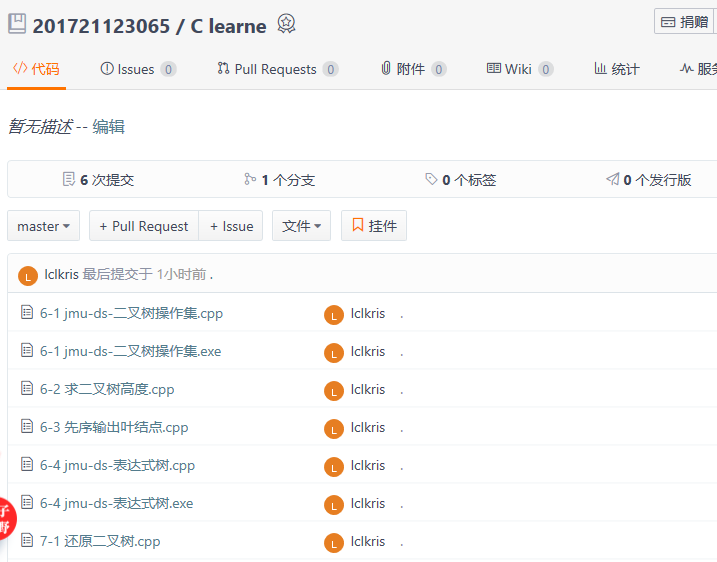posted @ 2018-05-05 20:47  LCLkris  阅读(353)  评论(0编辑  收藏  举报